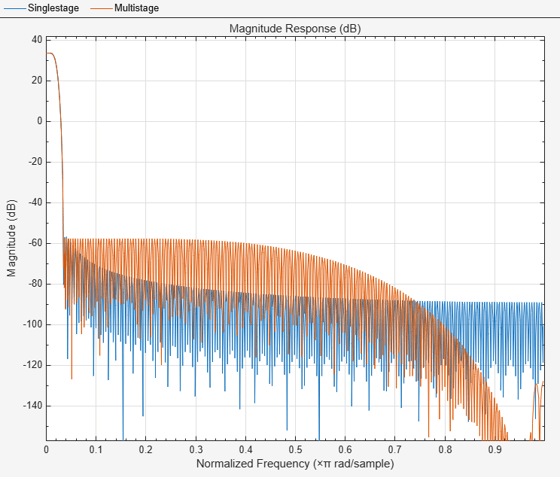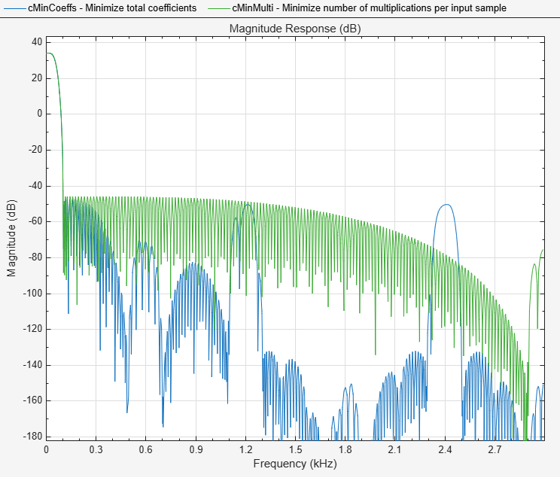# designMultistageInterpolator

Multistage interpolator design

## Syntax

``C = designMultistageInterpolator(L)``
``C = designMultistageInterpolator(L,Fs,TW)``
``C = designMultistageInterpolator(L,Fs,TW,Astop)``
``C = designMultistageInterpolator(___,Name,Value)``

## Description

````C = designMultistageInterpolator(L)` designs a multistage interpolator that has an overall interpolation factor of `L`. In order for `C` to be multistage, `L` must not be a prime number. For details, see Algorithms. The design process can take a while if `L` has many factors.```

example

````C = designMultistageInterpolator(L,Fs,TW)` designs a multistage interpolator with a sampling rate of `Fs` and a transition width of `TW`. Sampling rate in this case refers to the output sampling rate of the signal after the multistage interpolator.The multistage interpolator has a cutoff frequency of `Fs`/(2`L`).```

example

````C = designMultistageInterpolator(L,Fs,TW,Astop)` specifies a minimum attenuation of `Astop` dB for the resulting design.```

example

````C = designMultistageInterpolator(___,Name,Value)` specifies additional design parameters using one or more name-value pair arguments.Example: ```C = designMultistageInterpolator(48,48000,200,80,'NumStages','auto')``` designs a multistage interpolator with the least number of multiplications per input sample (MPIS).```

## Examples

collapse all

Design a single-stage interpolator using the `designMultirateFIR` function and a multistage interpolator using the `designMultistageInterpolator` function. Determine the efficiency of the two designs using the `cost` function. The implementation efficiency is characterized by two cost metrics: `NumCoefficients` and `MultiplicationsPerInputSample`.

Compute the cost of implementing both designs, and determine which design is more efficient. To make a comparison, design the filters such that their transition width is the same.

Initialization

Choose an interpolation factor of 48, input sample rate of 30.72 MHz, one-sided bandwidth of 100 kHz, and a stopband attenution of 90 dB.

```L = 48; Fin = 30.72e6; Astop = 90; BW = 1e5;```

Using the `designMultirateFIR` Function

Designing the interpolation filter using the `designMultirateFIR` function yields a single-stage design. Set the half-polyphase length to a finite integer, in this case 4.

```HalfPolyLength = 4; b = designMultirateFIR(L,1,HalfPolyLength,Astop); d = dsp.FIRInterpolator(L,b)```
```d = dsp.FIRInterpolator with properties: InterpolationFactor: 48 NumeratorSource: 'Property' Numerator: [0 -6.0692e-06 -1.4587e-05 -2.5889e-05 ... ] Show all properties ```

Compute the cost of implementing the interpolator. The interpolation filter requires 376 coefficients and 7 states. The number of multiplications per input sample and additions per input sample are 376 and 329, respectively.

`cost(d)`
```ans = struct with fields: NumCoefficients: 376 NumStates: 7 MultiplicationsPerInputSample: 376 AdditionsPerInputSample: 329 ```

Using the d`esignMultistageInterpolator` Function

Design a multistage interpolator with the same filter specifications as the single-stage design. Compute the transition width using the following relationship:

```Fc = Fin/(2*L); TW = 2*(Fc-BW);```

By default, the number of stages given by the `NumStages `argument is set to `'Auto'`, yielding an optimal design that tries to minimize the number of multiplications per input sample.

`c = designMultistageInterpolator(L,Fin,TW,Astop)`
```c = dsp.FilterCascade with properties: Stage1: [1x1 dsp.FIRInterpolator] Stage2: [1x1 dsp.FIRInterpolator] CloneStages: false ```

Calling the `info` function on `c` shows that the filter is implemented as a cascade of two `dsp.FIRInterpolator` objects with interpolation factors of 24 and 2, respectively.

Compute the cost of implementing the interpolator.

`cost(c)`
```ans = struct with fields: NumCoefficients: 184 NumStates: 12 MultiplicationsPerInputSample: 322 AdditionsPerInputSample: 275 ```

The `NumCoefficients` and the `MultiplicationsPerInputSample` parameters are lower for the two-stage filter designed by the `designMultistageInterpolator` function, making it more efficient.

Compare the magnitude response of both designs.

```hvft = fvtool(b,c); legend(hvft,'Single-stage','Multistage')```The magnitude response shows that the transition width of both the filters is the same, making the filters comparable. The `cost` function shows that implementing the multistage design is more efficient compared to implementing the single-stage design.

Using the `'design'` Option in the d`esignMultistageInterpolator` Function

The filter can be made even more efficient by setting the `'CostMethod'` argument of the `designMultistageInterpolator` function to `'design'`. By default, this argument is set to `'estimate'`.

In the `'design'` mode, the function designs each stage and computes the filter order. This yields an optimal design compared to the `'estimate'` mode, where the function estimates the filter order for each stage and designs the filter based on the estimate.

Note that the `'design'` option can take much longer compared to the `'estimate'` option.

`cOptimal = designMultistageInterpolator(L,Fin,TW,Astop,'CostMethod','design')`
```cOptimal = dsp.FilterCascade with properties: Stage1: [1x1 dsp.FIRInterpolator] Stage2: [1x1 dsp.FIRInterpolator] Stage3: [1x1 dsp.FIRInterpolator] CloneStages: false ```
`cost(cOptimal)`
```ans = struct with fields: NumCoefficients: 74 NumStates: 17 MultiplicationsPerInputSample: 296 AdditionsPerInputSample: 249 ```

Design an interpolator with an overall interpolation factor of 24 using the `designMultistageInterpolator` function. Design the filter in two configurations:

• Two-stage configuration - `NumStages` is set to 2.

• Auto configuration - `NumStages` is set to `'Auto'`. This configuration designs a filter with the lowest number of multiplications per input sample.

Compare the `cost` of implementing both the configurations.

Initialization

Choose an interpolation factor of 24, input sample rate of 6 kHz, stopband attenuation of 90 dB, and a transition width of 0.03$×$6000/2.

```L = 24; Fs = 6000; Astop = 90; TW = 0.03*Fs/2;```

Design the Filter

Design the two filters using the `designMultistageInterpolator` function.

`cAuto = designMultistageInterpolator(L,Fs,TW,Astop,'NumStages','Auto')`
```cAuto = dsp.FilterCascade with properties: Stage1: [1x1 dsp.FIRInterpolator] Stage2: [1x1 dsp.FIRInterpolator] Stage3: [1x1 dsp.FIRInterpolator] CloneStages: false ```
`cTwo = designMultistageInterpolator(L,Fs,TW,Astop,'NumStages',2)`
```cTwo = dsp.FilterCascade with properties: Stage1: [1x1 dsp.FIRInterpolator] Stage2: [1x1 dsp.FIRInterpolator] CloneStages: false ```

View the filter information using the `info` function. The `'Auto'` configuration designs a cascade of three FIR interpolators with interpolation factors 4, 3, and 2, respectively. The two-stage configuration designs a cascade of two FIR interpolators with interpolation factors 6 and 4, respectively.

Compare the Cost

Compare the cost of implementing the two designs using the `cost` function.

`cost(cAuto)`
```ans = struct with fields: NumCoefficients: 70 NumStates: 28 MultiplicationsPerInputSample: 190 AdditionsPerInputSample: 167 ```
`cost(cTwo)`
```ans = struct with fields: NumCoefficients: 98 NumStates: 22 MultiplicationsPerInputSample: 208 AdditionsPerInputSample: 185 ```

The `'Auto'` configuration interpolation filter yields a three-stage design that out-performs the two-stage design in terms of `NumCoefficients` and `MultiplicationsPerInputSample `metrics.

The filters in the multistage design satisfy the following conditions:

• The combined response must meet or exceed the given design specifications.

• The combined interpolation must equal the overall interpolation required.

For an overall interpolation factor of 50, there are several combinations of individual stages.

To obtain a design with the least number of total coefficients, set the `'MinTotalCoeffs'` argument to `true`.

```Astop = 80; L = 50; Fs = 6000; TW = 0.03*Fs/2; cMinCoeffs = designMultistageInterpolator(L,Fs,TW,Astop,'MinTotalCoeffs',true)```
```cMinCoeffs = dsp.FilterCascade with properties: Stage1: [1x1 dsp.FIRInterpolator] Stage2: [1x1 dsp.FIRInterpolator] Stage3: [1x1 dsp.FIRInterpolator] CloneStages: false ```
`cost(cMinCoeffs)`
```ans = struct with fields: NumCoefficients: 58 NumStates: 18 MultiplicationsPerInputSample: 306 AdditionsPerInputSample: 257 ```

To obtain the design with the lowest number of multiplications per input sample, set `'NumStages'` to '`auto'`.

`cMinMulti = designMultistageInterpolator(L,Fs,TW,Astop,'NumStages','auto')`
```cMinMulti = dsp.FilterCascade with properties: Stage1: [1x1 dsp.FIRInterpolator] Stage2: [1x1 dsp.FIRInterpolator] CloneStages: false ```
`cost(cMinMulti)`
```ans = struct with fields: NumCoefficients: 156 NumStates: 9 MultiplicationsPerInputSample: 252 AdditionsPerInputSample: 203 ```

Compare the magnitude response of both the filters using `fvtool`. Both filters have the same transition-band behavior and a stopband attenuation that is below 80 dB.

```hvft = fvtool(cMinCoeffs,cMinMulti); legend(hvft,'Minimize total coefficients','Minimize number of multiplications per input sample')```## Input Arguments

collapse all

Overall interpolation factor, specified as a positive integer greater than one. In order for `C` to be multistage, `L` must not be a prime number. For details, see Algorithms.

Data Types: `single` | `double` | `int8` | `int16` | `int32` | `int64` | `uint8` | `uint16` | `uint32` | `uint64`

Sampling rate of the output signal after the multistage interpolator, specified as a positive real scalar. If not specified, `Fs` defaults to 48,000 Hz. The multistage interpolator has a cutoff frequency of `Fs`/(2`L`).

Data Types: `single` | `double` | `int8` | `int16` | `int32` | `int64` | `uint8` | `uint16` | `uint32` | `uint64`

Transition width, specified as a positive real scalar less than `Fs`/`L`. If not specified, `TW` defaults to 0.2×`Fs`/`L`. Transition width must be less than `Fs`/`L`.

Data Types: `single` | `double` | `int8` | `int16` | `int32` | `int64` | `uint8` | `uint16` | `uint32` | `uint64`

Minimum stopband attenuation for the resulting design, specified as a positive real scalar.

Data Types: `single` | `double` | `int8` | `int16` | `int32` | `int64` | `uint8` | `uint16` | `uint32` | `uint64`

### Name-Value Arguments

Specify optional pairs of arguments as `Name1=Value1,...,NameN=ValueN`, where `Name` is the argument name and `Value` is the corresponding value. Name-value arguments must appear after other arguments, but the order of the pairs does not matter.

Before R2021a, use commas to separate each name and value, and enclose `Name` in quotes.

Example: ```C = designMultistageInterpolator(48,48000,200,80,'NumStages','auto')``` designs a multistage interpolator with the lowest number of multiplications per input sample.

Number of interpolator stages, specified as a positive integer. If set to `'auto'`, the design algorithm determines the number of stages that result in the lowest number of multiplications per input sample. If specified as a positive integer, N, the overall interpolation factor, `L`, must be able to factor into at least N factors, not counting `1` or `L` as factors.

Data Types: `single` | `double` | `int8` | `int16` | `int32` | `int64` | `uint8` | `uint16` | `uint32` | `uint64`

When `false`, the design algorithm minimizes the number of multiplications per input sample. When `true`, the design algorithm minimizes the total number of coefficients.

Data Types: `logical`

Cost computation method, specified as either:

• `'estimate'` –– The function estimates the filter order required for each stage and designs the filter based on the estimate. This method is faster than `'design'`, but can lead to suboptimal designs.

• `'design'` –– The function designs each stage and computes the filter order.

Data Types: `char`

Tolerance, specified as a positive scalar. The tolerance is used to determine the multistage configuration with the least number of MPIS. When multiple configurations result in the same lowest MPIS within the tolerance specified, the configuration that yields the lowest number of coefficients overall is chosen. To view the total number of coefficients and MPIS for a specific filter, use the `cost` function.

Data Types: `single` | `double` | `int8` | `int16` | `int32` | `int64` | `uint8` | `uint16` | `uint32` | `uint64`

## Output Arguments

collapse all

Designed filter, returned as a `dsp.FilterCascade` System object™. The filter is a cascade of the multiple stages designed by the function. The number of stages is determined by the `'NumStages'` argument.

To get information about each filter stage, call the `info` function on the `C` argument.

## Algorithms

The overall interpolation factor is split into smaller factors with each factor being the interpolation factor of the corresponding individual stage. The combined interpolation of all the individual stages must equal the overall interpolation. The combined response must meet or exceed the given design specifications.

The function determines the number of interpolator stages through the `'NumStages'` argument. The sequence of stages is determined based on the implementation cost. By default, `'NumStages'` is set to `'auto'`, resulting in a sequence that gives the lowest number of MPIS. When multiple configurations result in the same lowest MPIS within the tolerance specified, the configuration that yields the lowest number of coefficients overall is chosen. If `'MinTotalCoeffs'` is set to `true`, the function determines the sequence that requires the lowest number of total coefficients.

By default, the `'CostMethod'` is set to `'estimate'`. In this mode, the function estimates the filter order required for each stage and designs the filter based on the estimate. This method is faster but can lead to suboptimal designs. For an optimal design, set `'CostMethod'` to `'design'`. In this mode, the function designs each stage and computes the filter order.

## Version History

Introduced in R2018b